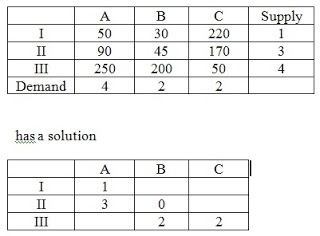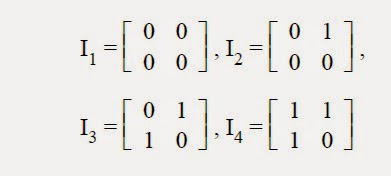11. The following transportation problem has a solution given below it. The solution of a given transportation problem isa. infeasible solution b. optimum solution c. non-optimum solution d. unbounded solution

 12. You are given four images represented as follows. The value of entropy is maximum for imagea. I1 b. I2 c. I3 d. I4